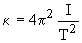# Finding torsion constant of the wire?

jazzymanstan

## Homework Statement

A torsional pendulum consists of a disk of mass 450 g and radius 3.5 cm, hanging from a wire. If the disk is rotated through an angle of 45o and released from rest and oscillates with a frequency of 2.5 Hz, what is the torsion constant of the wire?
• A :

1.7 x 10-3 N-m/rad

• B :

• C :

• D :

• E :

## Homework Equations

Torque = -k(torsion constant) * theta
Torque = r * F*sin theta

## The Attempt at a Solution

So i had to google "torsion constant equation" because i couldn't find an equation in my book so im hoping this is the right equation. Then i used the second torque equation to find torque. I did T = .035m * (.45kg * 9.8(gravity))*sin(45) = .10914.

Then i plugged the answer for T into the first equation T = -k *theta. So .10914 = -k * (pi/4), i converted 45 degrees to pi/4 radians. Then I divided .10914/(pi/4) = .13896.

So my overall answer was .13896. This is not one the options above so i know I did not do something correctly. I'm almost positive its from not having the right equations for the questions.

Advice would be great, thanks everyone!

## Answers and Replies

jazzymanstan
i solved it myself but i will leave it here for other people if they need help. ignoring all my work above, it is best to use equation. Then you use the appropriate inertia equation, which in this problem you'll use I = .5*m*r^2. And then for T, use T = 1/f. Plug those equations into the original equation, and then plug the numbers in for the correct variables, and you should get the right answer. (mine was .068 N-m/rad)